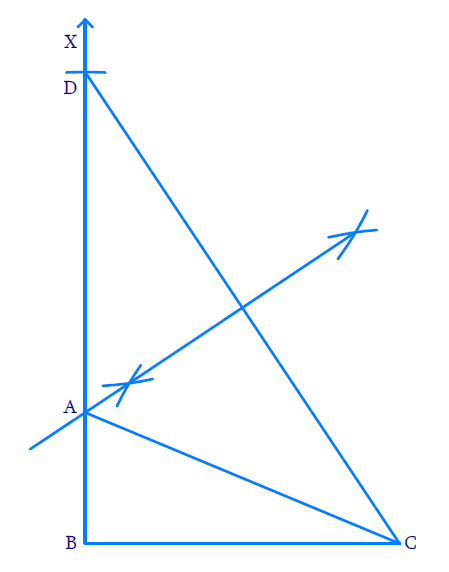In the verge of coronavirus pandemic, we are providing FREE access to our entire Online Curriculum to ensure Learning Doesn't STOP!

# Ex.11.2 Q5 Constructions Solution - NCERT Maths Class 9

Go back to  'Ex.11.2'

## Question

Construct a right triangle whose base is $$12\;\rm{cm}$$  and sum of its hypotenuse and other side is $$18\;\rm{cm}$$

Video Solution
Constructions
Ex 11.2 | Question 5

## Text Solution

Steps of Construction:(i) Draw the base $$BC=12\;\rm{cm}$$.

(ii) At the point $$B$$, make an angle $$CBX=90^\circ$$ using a protractor.

(iii) Cut a line segment $$BD=18\;\rm{cm}$$ from the ray $$BX$$.

(iv) Join $$DC$$

(v) With $$D$$ and $$C$$ as the centres and radius greater than half of $$DC$$ draw arcs on either side of the line to intersect each other. Join the intersecting points and extend the perpendicular bisector to meet $$BD$$ at $$A$$.

(vi) Join $$A$$ and $$C$$$$ABC$$ is the required right-angled triangle

Learn from the best math teachers and top your exams

• Live one on one classroom and doubt clearing
• Practice worksheets in and after class for conceptual clarity
• Personalized curriculum to keep up with school